Bookkeeping Skills Pre-Assessment part 2

Please give yourself an estimated amount of time of 7 minutes to take this assessment.

The use of calculators is prohibited while taking this assessment.

1 / 20

Category: Bookkeeping Skills Pre-Assessment Part 2

1) Each table contains ONE wrong number. Any of the numbers or totals may be wrong. To find the wrong number, check each column to see which one does not add correctly. Then check each row to see which one does not add correctly. You will find an intersection with the one wrong number. Please type the numerical value of the wrong number in the box below.2 / 20

Category: Bookkeeping Skills Pre-Assessment Part 2

2) Each table contains ONE wrong number. Any of the numbers or totals may be wrong. To find the wrong number, check each column to see which one does not add correctly. Then check each row to see which one does not add correctly. You will find an intersection with the one wrong number. Please type the numerical value of the wrong number in the box below.3 / 20

Category: Bookkeeping Skills Pre-Assessment Part 2

3) Each table contains ONE wrong number. Any of the numbers or totals may be wrong. To find the wrong number, check each column to see which one does not add correctly. Then check each row to see which one does not add correctly. You will find an intersection with the one wrong number. Please type the numerical value of the wrong number in the box below.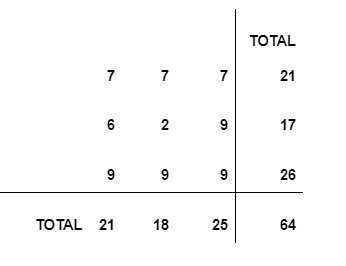4 / 20

Category: Bookkeeping Skills Pre-Assessment Part 2

4) Each table contains ONE wrong number. Any of the numbers or totals may be wrong. To find the wrong number, check each column to see which one does not add correctly. Then check each row to see which one does not add correctly. You will find an intersection with the one wrong number. Please type the numerical value of the wrong number in the box below.5 / 20

Category: Bookkeeping Skills Pre-Assessment Part 2

5) Each table contains ONE wrong number. Any of the numbers or totals may be wrong. To find the wrong number, check each column to see which one does not add correctly. Then check each row to see which one does not add correctly. You will find an intersection with the one wrong number. Please type the numerical value of the wrong number in the box below.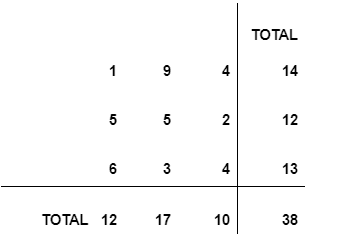6 / 20

Category: Bookkeeping Skills Pre-Assessment Part 2

6) Each table contains ONE wrong number. Any of the numbers or totals may be wrong. To find the wrong number, check each column to see which one does not add correctly. Then check each row to see which one does not add correctly. You will find an intersection with the one wrong number. Please type the numerical value of the wrong number in the box below.7 / 20

Category: Bookkeeping Skills Pre-Assessment Part 2

7) Each table contains ONE wrong number. Any of the numbers or totals may be wrong. To find the wrong number, check each column to see which one does not add correctly. Then check each row to see which one does not add correctly. You will find an intersection with the one wrong number. Please type the numerical value of the wrong number in the box below.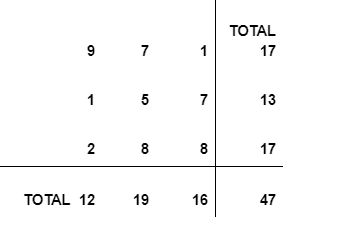8 / 20

Category: Bookkeeping Skills Pre-Assessment Part 2

8) Each table contains ONE wrong number. Any of the numbers or totals may be wrong. To find the wrong number, check each column to see which one does not add correctly. Then check each row to see which one does not add correctly. You will find an intersection with the one wrong number. Please type the numerical value of the wrong number in the box below.9 / 20

Category: Bookkeeping Skills Pre-Assessment Part 2

9) Each table contains ONE wrong number. Any of the numbers or totals may be wrong. To find the wrong number, check each column to see which one does not add correctly. Then check each row to see which one does not add correctly. You will find an intersection with the one wrong number. Please type the numerical value of the wrong number in the box below.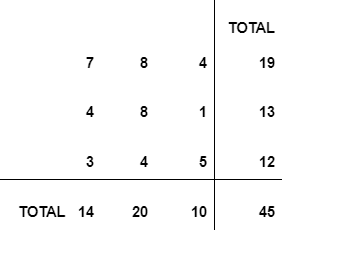10 / 20

Category: Bookkeeping Skills Pre-Assessment Part 2

10) Each table contains ONE wrong number. Any of the numbers or totals may be wrong. To find the wrong number, check each column to see which one does not add correctly. Then check each row to see which one does not add correctly. You will find an intersection with the one wrong number. Please type the numerical value of the wrong number in the box below.11 / 20

Category: Bookkeeping Skills Pre-Assessment Part 2

11) Each table contains ONE wrong number. Any of the numbers or totals may be wrong. To find the wrong number, check each column to see which one does not add correctly. Then check each row to see which one does not add correctly. You will find an intersection with the one wrong number. Please type the numerical value of the wrong number in the box below.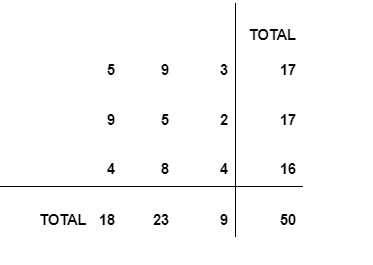12 / 20

Category: Bookkeeping Skills Pre-Assessment Part 2

12) Each table contains ONE wrong number. Any of the numbers or totals may be wrong. To find the wrong number, check each column to see which one does not add correctly. Then check each row to see which one does not add correctly. You will find an intersection with the one wrong number. Please type the numerical value of the wrong number in the box below.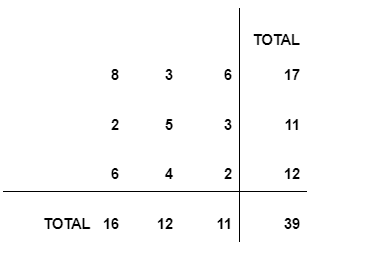13 / 20

Category: Bookkeeping Skills Pre-Assessment Part 2

13) Each table contains ONE wrong number. Any of the numbers or totals may be wrong. To find the wrong number, check each column to see which one does not add correctly. Then check each row to see which one does not add correctly. You will find an intersection with the one wrong number. Please type the numerical value of the wrong number in the box below.14 / 20

Category: Bookkeeping Skills Pre-Assessment Part 2

14) Each table contains ONE wrong number. Any of the numbers or totals may be wrong. To find the wrong number, check each column to see which one does not add correctly. Then check each row to see which one does not add correctly. You will find an intersection with the one wrong number. Please type the numerical value of the wrong number in the box below.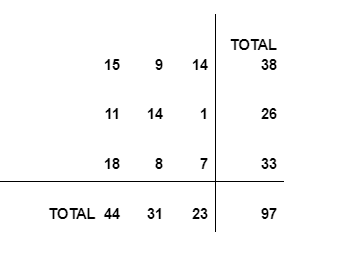15 / 20

Category: Bookkeeping Skills Pre-Assessment Part 2

15) Each table contains ONE wrong number. Any of the numbers or totals may be wrong. To find the wrong number, check each column to see which one does not add correctly. Then check each row to see which one does not add correctly. You will find an intersection with the one wrong number. Please type the numerical value of the wrong number in the box below.16 / 20

Category: Bookkeeping Skills Pre-Assessment Part 2

16) Each table contains ONE wrong number. Any of the numbers or totals may be wrong. To find the wrong number, check each column to see which one does not add correctly. Then check each row to see which one does not add correctly. You will find an intersection with the one wrong number. Please type the numerical value of the wrong number in the box below.17 / 20

Category: Bookkeeping Skills Pre-Assessment Part 2

17) Each table contains ONE wrong number. Any of the numbers or totals may be wrong. To find the wrong number, check each column to see which one does not add correctly. Then check each row to see which one does not add correctly. You will find an intersection with the one wrong number. Please type the numerical value of the wrong number in the box below.18 / 20

Category: Bookkeeping Skills Pre-Assessment Part 2

18) Each table contains ONE wrong number. Any of the numbers or totals may be wrong. To find the wrong number, check each column to see which one does not add correctly. Then check each row to see which one does not add correctly. You will find an intersection with the one wrong number. Please type the numerical value of the wrong number in the box below.19 / 20

Category: Bookkeeping Skills Pre-Assessment Part 2

19) Each table contains ONE wrong number. Any of the numbers or totals may be wrong. To find the wrong number, check each column to see which one does not add correctly. Then check each row to see which one does not add correctly. You will find an intersection with the one wrong number. Please type the numerical value of the wrong number in the box below.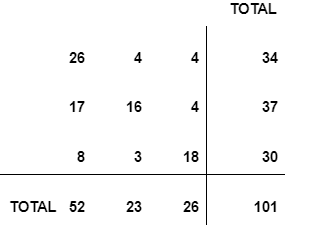20 / 20

Category: Bookkeeping Skills Pre-Assessment Part 2

20) Each table contains ONE wrong number. Any of the numbers or totals may be wrong. To find the wrong number, check each column to see which one does not add correctly. Then check each row to see which one does not add correctly. You will find an intersection with the one wrong number. Please type the numerical value of the wrong number in the box below.Your results have been submitted. Give all feedback for this assessment to Hiring@beardenstroup.com